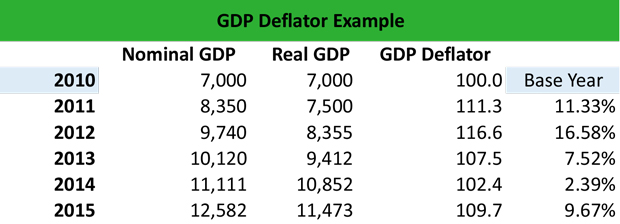# What is a GDP Deflator?

Definition: The GDP deflator, also known as the implicit price deflator, measures the impact of inflation on the gross domestic product during a specified period, usually a year.

## What Does GDP Deflator Mean?

What is the definition of GDP deflator? The GDP price deflator takes into consideration both the nominal GDP and the real GDP of an economy. The nominal GDP represents the value of the finished goods and services that an economyhas produced, unadjusted for inflation, whereas the real GDP represents the value of the finished goods and services that an economy has produced, adjusted for inflation.

Therefore, if there was no inflation involved, the nominal GDP would equal the real GDP. The GDP price inflator calculates the impact of inflation on the finished goods and products by converting an economy’s output into current prices, thereby demonstrating the impact of inflation on the GDP change.

Let’s look at an example.

## Example

To calculate the GDP price deflator formula, we need to know the nominal GDP and the real GDP. In the following example, 2010 is the base year. Then, every year we calculate the GDP deflator using the formula: GDP price deflator = Nominal GDP / Real GDP x 100

Therefore:1. 2010: 7,000 / 7,000 = 100.0
2. 2011: 8,350 / 7,500 = 111.3
3. 2012: 9,740 / 8,355 = 116.6
4. 2013: 10,120 / 9,412 = 107.5
5. 2014: 11,111 / 10,852 = 102.4
6. 2015: 12,582 / 11,473 = 109.7

Notice that in 2013 and 2014, the GDP price deflator decreases. This means that the increase in the aggregate level of prices is smaller in 2013 and in 2014 compared to the base year 2010. This is how the GDP deflator indicates the impact of inflation of the GDP, measuring the price inflation or deflation compared to the base year.

## Summary Definition

Define Gross Domestic Produce Deflator: GDP price deflator means a metric that is used to gauge the effects of inflation on a country’s output.